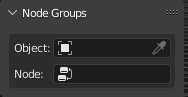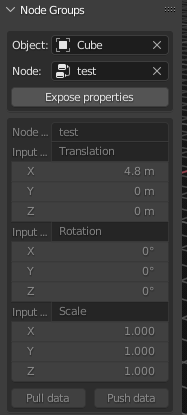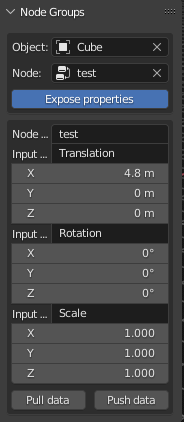# Creating Procedural Function Inputs for Panel UI

Hello! Currently I am working on a Python Script that should get inputs from a modifier that is based on exposed Geometry nodes, and then create input buttons based on them.

The Problem:
I have my list from the Geometry nodes, however I cannot seem to get a proper Function Input Reference. I am using col.prop(insert reference area here, insert function input reference). And I just cannot seem to get it to work with procedurally created inputs. They don’t seem to exist anywhere that I can access.

Talking to a more experienced friend he said that modifiers of geometry nodes are an instance, so that could be why I am having so much trouble accessing them.

Has anyone else experienced this kind of thing before, or have any idea of what to do?

Thank you!

Here is the code. I have been trying to find a solution and messing with it a lot. It does have multiple classes, but these are really the only relevant ones currently. The For loop under Draw is the main place of the difficulty.

``````class My_Properties(bpy.types.PropertyGroup):

global list_of_variables
list_of_var = []
my_string : bpy.props.StringProperty(name= "Enter Text")
my_amount : bpy.props.IntProperty(name= "Amount", soft_min= 0, soft_max= 1000, default= 1)

#modifier = bpy.context.scene.objects.modifiers["GeometryNodes"]

'''for input in modifier.node_group.inputs:
input : bpy.props.IntProperty(name= input, soft_min= 0, soft_max= 1000, default= 1)
list_of_var.append(input.name)'''

def create_definitions(self, variable_name):
#exec(f'variable_name : bpy.props.IntProperty(name= variable_name, soft_min= 0, soft_max= 1000, default= 1)')
variable_name : bpy.props.IntProperty(name= variable_name, soft_min= 0, soft_max= 1000, default= 1)
return variable_name

#Creating a panel for the User Interface
class PROCEDURAL_PT_panel(bpy.types.Panel):
bl_label = "Procedural Item"
bl_idname = "PROCEDURAL_PT_panel"
bl_space_type = 'VIEW_3D'
bl_region_type = 'UI'
bl_category = 'Procedural'

# Changable parameters
sliders_boolean = False
list_of_inputs = []

def draw(self, context):

layout = self.layout
scene = context.scene
mytool = scene.my_tool

global variables_generated
global list_of_variables

col = layout.column(align=True)
row = col.row(align=True)
col.operator(procedural.bl_idname, text = "Generate Object")

row = col.row(align=True)
amount_of_object = row.prop(mytool, "my_amount")

col = layout.column(align=True)
row = col.row(align=True)

col.operator(ui_def.bl_idname, text = "Generate Parameters")

modifier = bpy.context.active_object.modifiers["GeometryNodes"]

for input in modifier.node_group.inputs:
col.label(text=input.name)
input : bpy.props.IntProperty(name= input.name, soft_min= 0, soft_max= 1000, default= 1)
col.prop(modifier.node_group.inputs, input.name)

#Make these inputs
''' if(len(ui_def.input_list) > 0):

for a in ui_def.input_list:
if(variables_generated == False):
a : bpy.props.IntProperty(name= variable_name, soft_min= 0, soft_max= 1000, default= 1)
#list_of_variables.append(mytool.create_definitions(a))
#mytool.create_definitions(a)

row = col.row(align=True)
row.prop(self, a)
#row.prop(bpy.props, list_of_variables)
variables_generated = True

#row.prop(context.object, str(a), slider=True, text = str(a))
#self.list_of_inputs.append(a)'''

def invoke(self, context):
player_input = bpy.props.FloatProperty(name = "Enter Text:", default = 1)
return player_input

#Registering Classes
classes = (
PROCEDURAL_PT_panel,
procedural,
ui_def,
My_Properties
)

def register():
"""Register classes, properties"""
for cls in classes:
utils.make_annotations(cls)
bpy.utils.register_class(cls)
bpy.types.Scene.my_tool = bpy.props.PointerProperty(type= My_Properties)

def unregister():
"""Unregister classes, properties"""
for cls in reversed(classes):
bpy.utils.unregister_class(cls)
del bpy.types.Scene.my_tool

if __name__ == "__main__":
register()
``````

Not sure what your overall end goal is but I’ve thrown together a sample panel that may help get you on the right path.

# Edit

(15-April) Modified script to push or pull data from object modifier stack.

# Panel Options:The object selection uses a pointer and poll filter to select ‘MESH’ type objects (which can potentially have a geometry node modifer). Note this selection does not change the active or selected object in the scene and may cause confusion.

The node selection box uses a pointer and a poll filter to allow you to select any geometry node found in the current session of blender `bpy.data.node_groups`.

Once a valid combination (object that actually uses the node in its modifier stack) is selected the remainder of the panel will be displayed.The expose properties boolean simply toggles between enabling or disabling access to the lower portion of the panel.

# Panel Operators:

The Pull Data operator will locate the existing values in the modifier stack and update the panel with those values. (As mentioned above the selected object in the drop down may not be your active object)

The Push Data operator sends the values from the panel to the objects modifier stack and forces an object and scene update. (Known bug https://developer.blender.org/T87006)

# Script:

``````import bpy

def update_data(context, direction):
my_props = context.scene.test_pg
mod = [
mod for mod in my_props.my_obj.modifiers
if mod.type == 'NODES' and mod.node_group.name == my_props.my_node.name]
for input in mod.node_group.inputs:
if 'default_value' in input.bl_rna.properties:
data_path = mod[input.identifier]
try:
for num, i in enumerate(data_path):
ng_name = mod.node_group.name
ng = bpy.data.node_groups.get(ng_name)
ip_node = [
node for node in ng.nodes
if node.type == 'GROUP_INPUT']
if direction == "push":  # push from panel to mod stack
mod[input.identifier][num] = ip_node.outputs[input.name].default_value[num]
else:  # pull from mod stack to panel
ip_node.outputs[input.name].default_value[num] = mod[input.identifier][num]
except TypeError:
ng_name = mod.node_group.name
ng = bpy.data.node_groups.get(ng_name)
ip_node = [
node for node in ng.nodes
if node.type == 'GROUP_INPUT']
if direction == "push":  # push from panel to mod stack
mod[input.identifier] = ip_node.outputs[input.name].default_value
else:  # pull from mod stack to panel
ip_node.outputs[input.name].default_value = mod[input.identifier]

class OBJECT_OT_push_to_geo_mod(bpy.types.Operator):
'''Push panel data to modifier stack for selected object'''
bl_idname = 'object.push_to_geo_node'
bl_label = "Push data"

def execute(self, context):
my_props = context.scene.test_pg
update_data(context, direction="push")
# Known bug https://developer.blender.org/T87006
my_props.my_obj.update_tag()
context.scene.frame_set(context.scene.frame_current+1)
context.scene.frame_set(context.scene.frame_current-1)
return {'FINISHED'}

class OBJECT_OT_pull_from_geo_mod(bpy.types.Operator):
'''Pull data from modifier stack for selected object'''
bl_idname = 'object.pull_from_geo_mod'
bl_label = "Pull data"

def execute(self, context):
update_data(context, direction="pull")
return {'FINISHED'}

class VIEW3D_PT_test_ng(bpy.types.Panel):
bl_space_type = 'VIEW_3D'
bl_region_type = 'UI'
bl_category = "Testing"
bl_idname = "VIEW3D_PT_test_ng"
bl_label = "Node Groups"

def draw(self, context):
my_props = context.scene.test_pg
layout = self.layout
box = layout.box()
col = box.column(align=True)
col.enabled = True
col.prop(my_props, "my_obj", text="Object")
col.separator()
col.prop(my_props, "my_node", text="Node")
if not my_props.my_obj:
return
obj_mod_grps = [
mod.node_group.name for mod in my_props.my_obj.modifiers
if mod.type == 'NODES']
if my_props.my_node and my_props.my_node.name not in obj_mod_grps:
col.label(text=
f"{my_props.my_node.name} not used by {my_props.my_obj.name}")
return
col.separator()
if not my_props.my_node:
return
col.prop(my_props, "scn_var", text="Expose properties", toggle=1)

box = layout.box()
col = box.column(align=True)
col.enabled = my_props.scn_var
col.prop(my_props.my_node, "name", text="Node Group Name:")
# get the input node by type
ip_node = [
node for node in my_props.my_node.nodes
if node.type == 'GROUP_INPUT']
for ip in ip_node.outputs:
if 'default_value' in ip.bl_rna.properties:
col.label(text=f"{ip.name}")
col.prop(ip, "default_value", text="")
col.separator()
row = col.row()
row.operator("object.pull_from_geo_mod")
row.operator("object.push_to_geo_node")

def filter_geo_nodes(self, object):
return object.type == 'GEOMETRY'

def filter_obj(self, object):
return object.type == 'MESH'

class TEST_PG_props(bpy.types.PropertyGroup):
scn_var: bpy.props.BoolProperty(
name="scn_var",
description="Toggle Enable panel",
default=False,
)
my_node: bpy.props.PointerProperty(
type=bpy.types.NodeTree,
poll=filter_geo_nodes,)
my_obj: bpy.props.PointerProperty(
type=bpy.types.Object,
poll=filter_obj,)

classes = [
OBJECT_OT_push_to_geo_mod,
OBJECT_OT_pull_from_geo_mod,
VIEW3D_PT_test_ng,
TEST_PG_props,
]

def register():
for cls in classes:
bpy.utils.register_class(cls)
bpy.types.Scene.test_pg = bpy.props.PointerProperty(
type=TEST_PG_props)

def unregister():
for cls in classes:
bpy.utils.unregister_class(cls)
del bpy.types.Scene.test_pg

if __name__ == "__main__":
register()

``````

# Known Issue:

When attempting to push pull data from a string input field; if the original geometry node group did not set a default value the property is not properly instantiated and values will not be passed. You will need to set a value in the string field of the geometry module 1st then panel operations will work as expected.

# Geometry Node Group input & values:

When referencing the group input node (where people usually connect access points) the values that are changed are the output (right hand side) stored `bpy.data.node_groups['Geometry Nodes'].nodes['Group Input'].outputs`.

4 Likes

Thank you so much!!! This is amazing, and exactly what I was trying to do. Your code is very clear and readable, I appreciate so much!